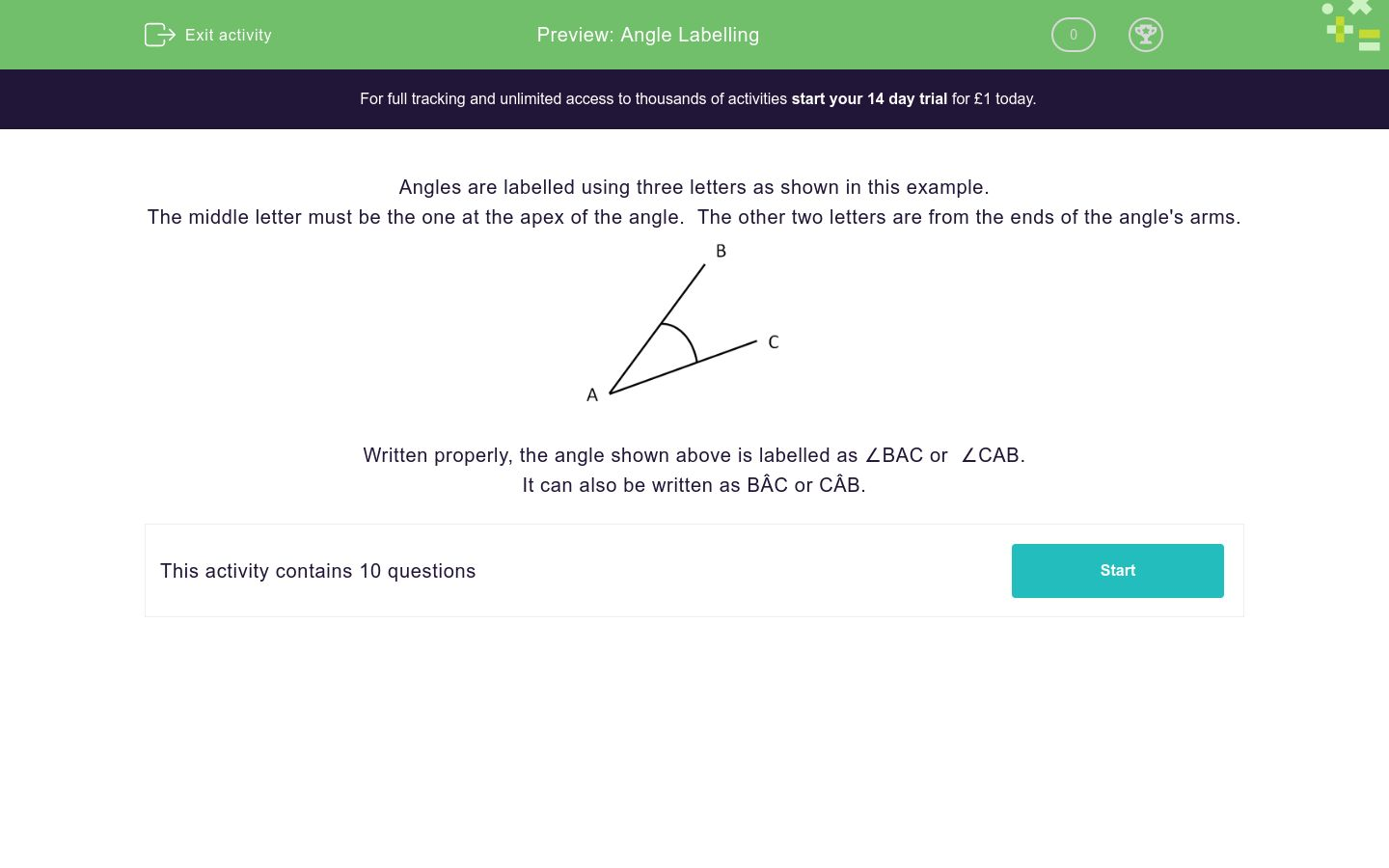# Angle Labelling

In this worksheet, students label angles using the correct three letters.Key stage:  KS 3

Curriculum topic:  Geometry and Measures

Curriculum subtopic:  Use Standard Conventions for Labelling Triangles

Difficulty level:### QUESTION 1 of 10

Angles are labelled using three letters as shown in this example.

The middle letter must be the one at the apex of the angle.  The other two letters are from the ends of the angle's arms.Written properly, the angle shown above is labelled as ∠BAC or  ∠CAB.

It can also be written as BÂC or CÂB.

Select all the ways of correctly labelling the angle shown in the diagram.∠ECB

∠EBC

∠BEC

∠BCE

∠CBE

∠CEB

Select all the ways of correctly labelling the angle shown in the diagram.∠ECD

ÊCD

∠DEC

∠DCE

CÊD

∠CED

Select all the ways of correctly labelling the angle shown in the diagram.∠CFG

CFG

∠FGC

∠CGF

∠GFC

∠F

Select all the ways of correctly labelling the angle shown in the diagram.∠FPH

PFH

∠PFH

∠PHF

∠HFP

∠F

Select all the ways of correctly labelling the angle shown in the diagram.∠ROP

POR

∠POR

PÔR

∠QOP

RÔP

Select all the ways of correctly labelling the angle shown in the diagram.∠RPI

PIR

∠PIQ

PÎQ

∠QIP

RÎQ

Select all the ways of correctly labelling the angle shown in the diagram.∠RPI

Q

∠RIQ

PÎQ

∠IQR

RÎQ

Select all the ways of correctly labelling the angle shown in the diagram.∠PAH

∠TAP

∠HAT

TÂH

∠ATH

PÂT

Select all the ways of correctly labelling the angle shown in the diagram.∠PAH

∠TAP

∠HAT

TÂH

∠ATH

PÂT

Select all the ways of correctly labelling the angle shown in the diagram.∠JIH

∠HIJ

∠JIF

JÎH

∠JKI

FÎJ

• Question 1

Select all the ways of correctly labelling the angle shown in the diagram.∠BEC
∠CEB
• Question 2

Select all the ways of correctly labelling the angle shown in the diagram.∠DEC
CÊD
∠CED
• Question 3

Select all the ways of correctly labelling the angle shown in the diagram.∠CFG
∠GFC
• Question 4

Select all the ways of correctly labelling the angle shown in the diagram.∠PFH
∠HFP
• Question 5

Select all the ways of correctly labelling the angle shown in the diagram.∠ROP
∠POR
PÔR
RÔP
• Question 6

Select all the ways of correctly labelling the angle shown in the diagram.∠PIQ
PÎQ
∠QIP
• Question 7

Select all the ways of correctly labelling the angle shown in the diagram.∠IQR
• Question 8

Select all the ways of correctly labelling the angle shown in the diagram.∠HAT
TÂH
• Question 9

Select all the ways of correctly labelling the angle shown in the diagram.∠TAP
PÂT
• Question 10

Select all the ways of correctly labelling the angle shown in the diagram.